# Money multiplier

The simple money multiplier or deposit multiplier measures how much money a certain deposit can create when the banking sector engages in fractional reserve banking. The money multiplier is an important concept for central banks. Central banks can also modify the money multiplier by changing the reserve requirements.

The multiplier is important both in macroeconomics and in banking. For macroeconomics it is important because it determines the money supply, which influences interest rates. For banking it is important because it has an impact on monetary policy and the banking sector’s stability.

On this page, we discuss the money multiplier definition, describe the money multiplier formula, and discuss a simple example of the multiplier effect. At the bottom of this page, we include an Excel spreadsheet that implements a money multiplier calculator.

## Money multiplier definition

What is the money multiplier effect? The multiplier effect follows naturally from banks’ loan origination process. In particular, when banks lend out money to borrowers, the banks typically lend out only a certain fraction and not the full amount of deposits their clients deposited with them. The fraction of deposits they continue to hold is used to meet withdrawals by banking clients. In fact, banks are legally required to maintain a certain fraction of the deposits for this purpose. This is requirement is called the reserve requirement or required reserve ratio.

The money that banks are not required to hold, is turned into loans.  That borrowed money in turn ends op on the deposit accounts of other clients. Again, the banks originate loans and are required to hold a certain fraction as reserves. This whole process of originating loans, these deposits flowing back to the banks and banks lending out a fraction of the money again is called fractional reserve banking.

The total amount of new deposits or new money that is created can be captured using the money multiplier formula. However, to calculate the money multiplier, we first need to determine the reserve ratio. We discuss the calculation of the reserve ratio below.

## Reserve ratio formula

How to calculate required reserve ratio? The reserve ratio or reserve requirement formula is defined as follows: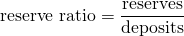Thus, to calculate the reserve ratio, we need balance sheet data of the bank for which we want to calculate the reserve ratio.Typically banks will hold reserves that just meet the reserve requirements set out by the central banks. This is because any excess deposits can be turned into loans, generating revenues. As an example, suppose the reserve requirement is 2%. Then a bank with $1 billion in deposits will hold$20 million on reserve.

## Money multiplier formula

Now that we have the reserve requirement, we can calculate the simple money multiplier. The simple money multiplier formula is the following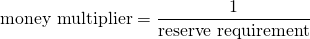The total amount of money that can be created for a given reserve requirement can also be calculated easily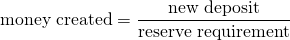## High-powered money and money multiplier

The new deposit is cash that is created by the central bank and is sometimes referred to as high-powered money. It is “high-powered” because it enables the banking sector to create a additional money through the multiplier effect. How this works, we discuss below.

## Money multiplier example

Now that we discussed money multiplier equation, we can implement a simple example. Suppose the central bank creates a \$1000 in high-powered money. The reserve requirement is 25%. What is the total amount of money that can be created?

Using the formulae above, we find that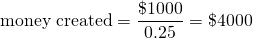and a multiplier of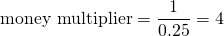This means that one dollar of excess deposits or reserves can lead to a four dollar increase in the money supply. From the example it is clear that the money supply is strongly dependent on the reserve requirement.

## Summary

We discussed the multiplier effect. The effect implies that an initial deposit of money can lead to multifold increase in the total money supply. The multiplier is used a lot in macroeconomics and banking. For example, central banks can set the reserve requirements to influence the money supply. Below we implement a money multiplier calculator in Excel that implements the above formulae.